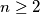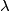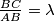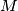### IMO Shortlist 2000 problem A5

Kvaliteta:
Avg: 0,0
Težina:
Avg: 8,0
Let$n \geq 2$ be a positive integer and$\lambda$ a positive real number. Initially there are$n$ fleas on a horizontal line, not all at the same point. We define a move as choosing two fleas at some points$A$ and$B$, with$A$ to the left of$B$, and letting the flea from$A$ jump over the flea from$B$ to the point$C$ so that$\frac {BC}{AB} = \lambda$.

Determine all values of$\lambda$ such that, for any point$M$ on the line and for any initial position of the$n$ fleas, there exists a sequence of moves that will take them all to the position right of$M$.
Izvor: Međunarodna matematička olimpijada, shortlist 2000